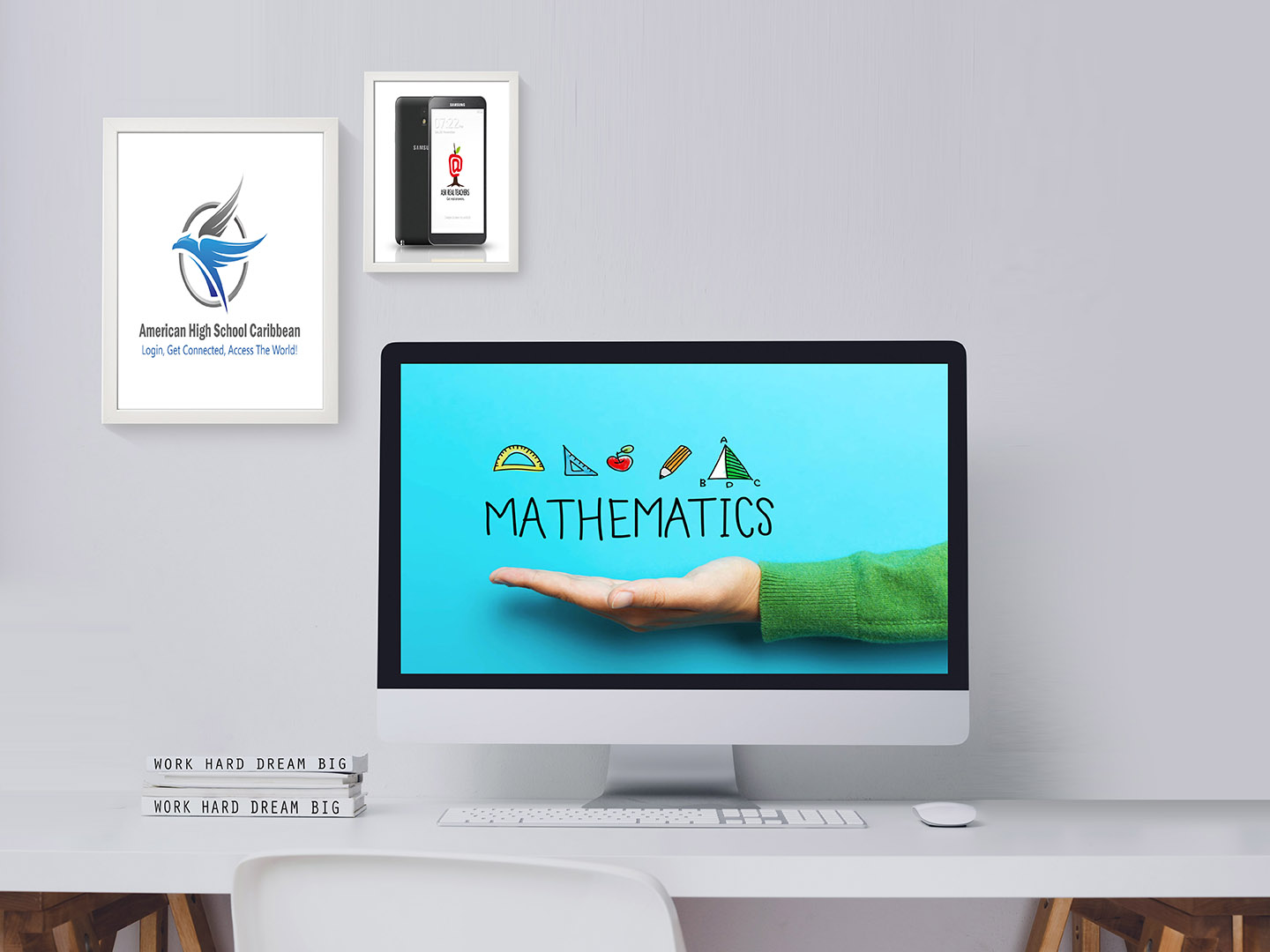## 2021 - 2022 Bahamas Virtual School Course List## Middle School Math

The sixth-grade math curriculum is designed for students to master addition, subtraction, multiplication, and division of
whole numbers, decimals, and fractions; graphing and statistics; measurement and geometry; ratio, proportion, and probability; percent; area and volume; and integers.

The seventh-grade curriculum contains strong math content with real-life connections. This course includes the basic study of exponents, rounding, place value, metrics, order of operations, using various graphs, adding, subtracting, multiplication, decimals.

This course includes the primary study of percent applications, exponents, scientific notation, metrics, multi-step problems,
lowest common multiples, most significant common factors, fractions, decimals, graphing, geometry, probability, statistics, and two variable equations in algebra.

## High School Math

##### Algebra I

This course is a study of the topics of Algebra I designed to develop the algebraic concepts and processes used to solve a variety of real-world mathematics problems. The content will include properties of the real number
system varied means for analyzing and expressing patterns.

##### Algebra 2

This course is designed to continue the study of algebra and to provide the foundation for applying these skills to other
mathematical and scientific fields. The content will include: the structure and properties of the complex number system;
sequences and series; relations; functions and graphs; varied solution strategies…

##### Geometry

The purpose of this course is to develop the geometric relationships and deductive strategies that can be used to solve a variety of real-world mathematical problems. The content will include Euclidean geometry of lines, planes, angles, triangles, construction and logic, properties of circles, polygons, right triangle..

##### Pre-Calcus

This course is designed to strengthen and extend the student’s knowledge of algebraic and trigonometric concepts and prepare the student for calculus. The content will include induction, symbolic logic, Boolean and matrix algebra, probability and statistics, elementary functions, and limits.

## WHAT ARE YOU WAITING FOR? ENROLL NOW!

Online High School Credit Recovery Programs and Courses
Complete your high school education on your terms in an accredited program on the Bahamas Virtual School platform. Study online at your own pace, guided by certified teachers.
SEE ALL
##### Contact Info
The BVS aims to provide an educational system that is technologically sound and competitive.
• #2 Pineapple Business Park, Airport, Industrial Park, Nassau, NP Bahamas
• +1 (242) 818-1418
• [email protected]